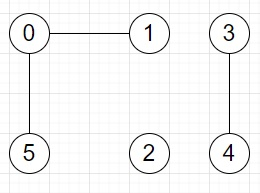# Program to find number of friend groups in a set of friends connections in Python

Suppose we have a a friends list, where friends[i] is a list of people i is friends with. The connection of friendships are two-way. And each person is friend with themselves and two people are in a friend group as long as there is some path of mutual friends connecting them. We have to find the total number of friend groups.

So, if the input is like friends = [[0, 1, 5],[1, 0],,[3, 4],[4, 3],[5, 0]], then the output will be 3, as The three friend groups are as below −To solve this, we will follow these steps −

• nodes := size of friends
• visited := a list of size same as nodes and fill with False
• ans := 0
• Define a function dfs() . This will take vertex, visited
• visited[vertex] := True
• for each nei in friends[vertex], do
• if visited[nei] is false, then
• dfs(nei, visited)
• From the main method, do the following −
• for i in range 0 to nodes, do
• if visited[i] is false, then
• dfs(i, visited)
• ans := ans + 1
• return ans

Let us see the following implementation to get better understanding −

## Example

Live Demo

class Solution:
def solve(self, friends):
nodes = len(friends)
visited = [False for _ in range(nodes)]
ans = 0
def dfs(vertex, visited): visited[vertex] = True
for nei in friends[vertex]:
if not visited[nei]:
dfs(nei, visited)
for i in range(nodes):
if not visited[i]:
dfs(i, visited)
ans += 1
return ans
ob = Solution()
friends = [ [0, 1, 5], [1, 0], , [3, 4], [4, 3], [5, 0] ]
print(ob.solve(friends))

## Input

[[0, 1, 5],
[1, 0],
,
[3, 4],
[4, 3],
[5, 0]
]

## Output

3